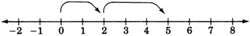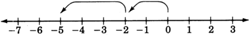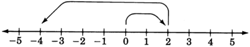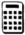# 3.3 Addition of signed numbers

 Page 1 / 1
This module is from Elementary Algebra by Denny Burzynski and Wade Ellis, Jr. The basic operations with real numbers are presented in this chapter. The concept of absolute value is discussed both geometrically and symbolically. The geometric presentation offers a visual understanding of the meaning of |x|. The symbolic presentation includes a literal explanation of how to use the definition. Negative exponents are developed, using reciprocals and the rules of exponents the student has already learned. Scientific notation is also included, using unique and real-life examples.Objectives of this module: be able to add numbers with like signs and unlike signs, understand addition with zero.

## Overview

• Addition of Numbers with Like Signs
• Addition with Zero
• Addition of Numbers with Unlike Signs

## Addition of numbers with like signs

Let us add the two positive numbers 2 and 3. We perform this addition on the number line as follows.

We begin at 0, the origin.
Since 2 is positive, we move 2 units to the right.
Since 3 is positive, we move 3 more units to the right.
We are now located at 5.
Thus, $2+3=5$ .Summarizing, we have

$\left(2\text{\hspace{0.17em}}\text{positive}\text{\hspace{0.17em}}\text{units}\right)+\left(3\text{\hspace{0.17em}}\text{positive}\text{\hspace{0.17em}}\text{units}\right)=\left(5\text{\hspace{0.17em}}\text{positive}\text{\hspace{0.17em}}\text{units}\right)$

Now let us add the two negative numbers $-2$ and $-3$ . We perform this addition on the number line as follows.

We begin at 0, the origin.
Since $-2$ is negative, we move 2 units to the left.
Since $-3$ is negative, we move 3 more units to the left.
We are now located at $-5$ .

Thus, $\left(-2\right)+\left(-3\right)=-5$ .Summarizing, we have

$\left(2\text{\hspace{0.17em}}\text{negative}\text{\hspace{0.17em}}\text{units}\right)+\left(3\text{\hspace{0.17em}}\text{negative}\text{\hspace{0.17em}}\text{units}\right)=\left(5\text{\hspace{0.17em}}\text{negative}\text{\hspace{0.17em}}\text{units}\right)$

These two examples suggest that

$\begin{array}{l}\left(\text{positive}\text{\hspace{0.17em}}\text{number}\right)\text{\hspace{0.17em}}+\text{\hspace{0.17em}}\left(\text{positive}\text{\hspace{0.17em}}\text{number}\right)\text{\hspace{0.17em}}=\text{\hspace{0.17em}}\left(\text{positive}\text{\hspace{0.17em}}\text{number}\right)\\ \left(\text{negative}\text{\hspace{0.17em}}\text{number}\right)\text{\hspace{0.17em}}+\text{\hspace{0.17em}}\left(\text{negative}\text{\hspace{0.17em}}\text{number}\right)\text{\hspace{0.17em}}=\text{\hspace{0.17em}}\left(\text{negative}\text{\hspace{0.17em}}\text{number}\right)\end{array}$

## Adding numbers with the same sign

To add two real numbers that have the same sign, add the absolute values of the numbers and associate the common sign with the sum.

## Sample set a

Find the sums.

$3+7$

$\begin{array}{l}\begin{array}{cc}& \text{\hspace{0.17em}}\text{\hspace{0.17em}}\text{\hspace{0.17em}}\text{\hspace{0.17em}}\text{\hspace{0.17em}}\text{\hspace{0.17em}}\text{\hspace{0.17em}}\text{\hspace{0.17em}}\text{\hspace{0.17em}}\text{\hspace{0.17em}}\text{\hspace{0.17em}}\text{\hspace{0.17em}}\text{\hspace{0.17em}}\text{\hspace{0.17em}}\text{\hspace{0.17em}}\text{\hspace{0.17em}}\text{\hspace{0.17em}}\text{\hspace{0.17em}}\text{\hspace{0.17em}}\text{\hspace{0.17em}}\text{\hspace{0.17em}}\text{\hspace{0.17em}}\text{\hspace{0.17em}}\text{\hspace{0.17em}}\text{\hspace{0.17em}}\text{\hspace{0.17em}}\text{\hspace{0.17em}}\text{\hspace{0.17em}}\text{\hspace{0.17em}}\text{\hspace{0.17em}}\text{\hspace{0.17em}}\text{\hspace{0.17em}}\text{Add}\text{\hspace{0.17em}}\text{these}\text{\hspace{0.17em}}\text{absolute}\text{\hspace{0.17em}}\text{values}\text{.}\end{array}\\ \begin{array}{cc}\begin{array}{l}|3|=3\\ |7|=7\end{array}\right\}& \text{\hspace{0.17em}}\text{\hspace{0.17em}}\text{\hspace{0.17em}}\text{\hspace{0.17em}}\text{\hspace{0.17em}}\text{\hspace{0.17em}}\text{\hspace{0.17em}}3+7=10\end{array}\\ \begin{array}{cc}& \text{\hspace{0.17em}}\text{\hspace{0.17em}}\text{\hspace{0.17em}}\text{\hspace{0.17em}}\text{\hspace{0.17em}}\text{\hspace{0.17em}}\text{\hspace{0.17em}}\text{\hspace{0.17em}}\text{\hspace{0.17em}}\text{\hspace{0.17em}}\text{\hspace{0.17em}}\text{\hspace{0.17em}}\text{\hspace{0.17em}}\text{\hspace{0.17em}}\text{\hspace{0.17em}}\text{\hspace{0.17em}}\text{\hspace{0.17em}}\text{\hspace{0.17em}}\text{\hspace{0.17em}}\text{\hspace{0.17em}}\text{\hspace{0.17em}}\text{\hspace{0.17em}}\text{\hspace{0.17em}}\text{\hspace{0.17em}}\text{\hspace{0.17em}}\text{\hspace{0.17em}}\text{\hspace{0.17em}}\text{\hspace{0.17em}}\text{\hspace{0.17em}}\text{\hspace{0.17em}}\text{\hspace{0.17em}}\text{\hspace{0.17em}}\text{The}\text{\hspace{0.17em}}\text{common}\text{\hspace{0.17em}}\text{sign}\text{\hspace{0.17em}}\text{is}\text{\hspace{0.17em}}\text{"+}\text{."}\end{array}\end{array}$

$3+7=+10\text{\hspace{0.17em}}\text{\hspace{0.17em}}\text{\hspace{0.17em}}\text{\hspace{0.17em}}\text{\hspace{0.17em}}\text{\hspace{0.17em}}\text{\hspace{0.17em}}\text{\hspace{0.17em}}\text{\hspace{0.17em}}\text{\hspace{0.17em}}\text{\hspace{0.17em}}\text{or}\text{\hspace{0.17em}}\text{\hspace{0.17em}}\text{\hspace{0.17em}}\text{\hspace{0.17em}}\text{\hspace{0.17em}}\text{\hspace{0.17em}}\text{\hspace{0.17em}}\text{\hspace{0.17em}}\text{\hspace{0.17em}}\text{\hspace{0.17em}}\text{\hspace{0.17em}}3+7=10$

$\left(-4\right)+\left(-9\right)$

$\begin{array}{l}\begin{array}{cc}& \text{\hspace{0.17em}}\text{\hspace{0.17em}}\text{\hspace{0.17em}}\text{\hspace{0.17em}}\text{\hspace{0.17em}}\text{\hspace{0.17em}}\text{\hspace{0.17em}}\text{\hspace{0.17em}}\text{\hspace{0.17em}}\text{\hspace{0.17em}}\text{\hspace{0.17em}}\text{\hspace{0.17em}}\text{\hspace{0.17em}}\text{\hspace{0.17em}}\text{\hspace{0.17em}}\text{\hspace{0.17em}}\text{\hspace{0.17em}}\text{\hspace{0.17em}}\text{\hspace{0.17em}}\text{\hspace{0.17em}}\text{\hspace{0.17em}}\text{\hspace{0.17em}}\text{\hspace{0.17em}}\text{\hspace{0.17em}}\text{\hspace{0.17em}}\text{\hspace{0.17em}}\text{\hspace{0.17em}}\text{\hspace{0.17em}}\text{\hspace{0.17em}}\text{\hspace{0.17em}}\text{\hspace{0.17em}}\text{\hspace{0.17em}}\text{\hspace{0.17em}}\text{\hspace{0.17em}}\text{\hspace{0.17em}}\text{Add}\text{\hspace{0.17em}}\text{these}\text{\hspace{0.17em}}\text{absolute}\text{\hspace{0.17em}}\text{values}\text{.}\end{array}\\ \begin{array}{cc}\begin{array}{l}|-4|=4\\ |-9|=9\end{array}\right\}& \text{\hspace{0.17em}}\text{\hspace{0.17em}}\text{\hspace{0.17em}}\text{\hspace{0.17em}}\text{\hspace{0.17em}}\text{\hspace{0.17em}}\text{\hspace{0.17em}}4+9=13\end{array}\\ \begin{array}{cc}& \text{\hspace{0.17em}}\text{\hspace{0.17em}}\text{\hspace{0.17em}}\text{\hspace{0.17em}}\text{\hspace{0.17em}}\text{\hspace{0.17em}}\text{\hspace{0.17em}}\text{\hspace{0.17em}}\text{\hspace{0.17em}}\text{\hspace{0.17em}}\text{\hspace{0.17em}}\text{\hspace{0.17em}}\text{\hspace{0.17em}}\text{\hspace{0.17em}}\text{\hspace{0.17em}}\text{\hspace{0.17em}}\text{\hspace{0.17em}}\text{\hspace{0.17em}}\text{\hspace{0.17em}}\text{\hspace{0.17em}}\text{\hspace{0.17em}}\text{\hspace{0.17em}}\text{\hspace{0.17em}}\text{\hspace{0.17em}}\text{\hspace{0.17em}}\text{\hspace{0.17em}}\text{\hspace{0.17em}}\text{\hspace{0.17em}}\text{\hspace{0.17em}}\text{\hspace{0.17em}}\text{\hspace{0.17em}}\text{\hspace{0.17em}}\text{\hspace{0.17em}}\text{\hspace{0.17em}}\text{\hspace{0.17em}}\text{The}\text{\hspace{0.17em}}\text{common}\text{\hspace{0.17em}}\text{sign}\text{\hspace{0.17em}}\text{is}\text{\hspace{0.17em}}\text{"}-\text{."}\end{array}\end{array}$

$\left(-4\right)+\left(-9\right)=-13$

## Practice set a

Find the sums.

$8+6$

14

$41+11$

52

$\left(-4\right)+\left(-8\right)$

$-12$

$\left(-36\right)+\left(-9\right)$

$-45$

$-14+\left(-20\right)$

$-34$

$-\frac{2}{3}+\left(-\frac{5}{3}\right)$

$-\frac{7}{3}$

$-2.8+\left(-4.6\right)$

$-7.4$

Notice that

## Addition with 0

$\begin{array}{l}\left(\text{0}\right)\text{\hspace{0.17em}}+\text{\hspace{0.17em}}\left(\text{a}\text{\hspace{0.17em}}\text{positive}\text{\hspace{0.17em}}\text{number}\right)\text{\hspace{0.17em}}=\text{\hspace{0.17em}}\left(\text{that}\text{\hspace{0.17em}}\text{same}\text{\hspace{0.17em}}\text{​}\text{positive}\text{\hspace{0.17em}}\text{number}\right)\\ \left(\text{0}\right)\text{\hspace{0.17em}}+\text{\hspace{0.17em}}\left(\text{a}\text{\hspace{0.17em}}\text{negative}\text{\hspace{0.17em}}\text{number}\right)\text{\hspace{0.17em}}=\text{\hspace{0.17em}}\left(\text{that}\text{\hspace{0.17em}}\text{same}\text{\hspace{0.17em}}\text{​}\text{negative}\text{\hspace{0.17em}}\text{number)}\end{array}$

## The additive identity is 0

Since adding 0 to a real number leaves that number unchanged, 0 is called the additive identity .

## Addition of numbers with unlike signs

Now let us perform the addition $2+\left(-6\right)$ . These two numbers have unlike signs. This type of addition can also be illustrated using the number line.

We begin at 0, the origin.
Since 2 is positive, we move 2 units to the right.
Since $-6$ is negative, we move, from the 2, 6 units to the left.
We are now located at $-4$ .A rule for adding two numbers that have unlike signs is suggested by noting that if the signs are disregarded, 4 can be obtained from 2 and 6 by subtracting 2 from 6. But 2 and 6 are precisely the absolute values of 2 and $-6$ . Also, notice that the sign of the number with the larger absolute value is negative and that the sign of the resulting sum is negative.

## Adding numbers with unlike signs

To add two real numbers that have unlike signs, subtract the smaller absolute value from the larger absolute value and associate the sign of the number with the larger absolute value with this difference.

## Sample set b

Find the following sums.

$7+\left(-2\right)$

$\begin{array}{cc}\underset{\begin{array}{l}\text{Larger}\text{\hspace{0.17em}}\text{absolute}\text{\hspace{0.17em}}\text{value}\text{.}\\ \text{Sign}\text{\hspace{0.17em}}\text{\hspace{0.17em}}\text{is}\text{\hspace{0.17em}}"+".\end{array}}{\underbrace{|7|=7}}\text{\hspace{0.17em}}\text{\hspace{0.17em}}\text{\hspace{0.17em}}& \underset{\text{Smaller}\text{\hspace{0.17em}}\text{absolute}\text{\hspace{0.17em}}\text{value}\text{.}}{\underbrace{|-2|=2}}\end{array}$

$\begin{array}{ll}\text{Subtract}\text{\hspace{0.17em}}\text{absolute}\text{\hspace{0.17em}}\text{values:}\hfill & 7-2=5.\hfill \\ \text{Attach}\text{\hspace{0.17em}}\text{the}\text{\hspace{0.17em}}\text{proper}\text{\hspace{0.17em}}\text{sign:}\hfill & "+".\hfill \end{array}$

$7+\left(-2\right)=+5\text{\hspace{0.17em}}\text{\hspace{0.17em}}\text{\hspace{0.17em}}\text{\hspace{0.17em}}\text{\hspace{0.17em}}\text{\hspace{0.17em}}\text{\hspace{0.17em}}\text{\hspace{0.17em}}\text{\hspace{0.17em}}\text{\hspace{0.17em}}\text{or}\text{\hspace{0.17em}}\text{\hspace{0.17em}}\text{\hspace{0.17em}}\text{\hspace{0.17em}}\text{\hspace{0.17em}}\text{\hspace{0.17em}}\text{\hspace{0.17em}}\text{\hspace{0.17em}}\text{\hspace{0.17em}}\text{\hspace{0.17em}}7+\left(-2\right)=5$

$3+\left(-11\right)$

$\begin{array}{cc}\underset{\text{Smaller}\text{\hspace{0.17em}}\text{absolute}\text{\hspace{0.17em}}\text{value}\text{.}}{\underbrace{|3|=3}}\text{\hspace{0.17em}}\text{\hspace{0.17em}}\text{\hspace{0.17em}}& \underset{\begin{array}{l}\text{Larger}\text{\hspace{0.17em}}\text{absolute}\text{\hspace{0.17em}}\text{value}\text{.}\\ \text{Sign}\text{\hspace{0.17em}}\text{is}\text{\hspace{0.17em}}"-".\end{array}}{\underbrace{|-11|=11}}\end{array}$

$\begin{array}{ll}\text{Subtract}\text{\hspace{0.17em}}\text{absolute}\text{\hspace{0.17em}}\text{values:}\hfill & 11-3=8.\hfill \\ \text{Attach}\text{\hspace{0.17em}}\text{the}\text{\hspace{0.17em}}\text{proper}\text{\hspace{0.17em}}\text{sign:}\hfill & "-".\hfill \end{array}$

$3+\left(-11\right)=-8$

The morning temperature on a winter's day in Lake Tahoe was $-12$ degrees. The afternoon temperature was 25 degrees warmer. What was the afternoon temperature?

We need to find $-12+25$ .

$\begin{array}{cc}\underset{\text{Smaller}\text{\hspace{0.17em}}\text{absolute}\text{\hspace{0.17em}}\text{value}\text{.}}{\underbrace{|-12|=12}}\text{\hspace{0.17em}}\text{\hspace{0.17em}}\text{\hspace{0.17em}}& \underset{\begin{array}{l}\text{Larger}\text{​}\text{\hspace{0.17em}}\text{absolute}\text{\hspace{0.17em}}\text{value}\text{.}\\ \text{Sign}\text{\hspace{0.17em}}\text{is}\text{\hspace{0.17em}}\text{"+"}\text{.}\end{array}}{\underbrace{|25|=25}}\end{array}$

$\begin{array}{ll}\text{Subtract}\text{\hspace{0.17em}}\text{absolute}\text{\hspace{0.17em}}\text{values:}\hfill & 25-12=13.\hfill \\ \text{Attach}\text{\hspace{0.17em}}\text{the}\text{\hspace{0.17em}}\text{proper}\text{\hspace{0.17em}}\text{sign:}\hfill & "+".\hfill \end{array}$

$-12+25=13$

Thus, the afternoon temperature is 13 degrees.Add $-147+84$ .                                                      Display Reads

$\begin{array}{l}\begin{array}{ccc}\text{Type}& \text{\hspace{0.17em}}\text{\hspace{0.17em}}\text{\hspace{0.17em}}\text{\hspace{0.17em}}\text{\hspace{0.17em}}147& \text{\hspace{0.17em}}\text{\hspace{0.17em}}\text{\hspace{0.17em}}\text{\hspace{0.17em}}\text{\hspace{0.17em}}\text{\hspace{0.17em}}\text{\hspace{0.17em}}\text{\hspace{0.17em}}147\end{array}\\ \begin{array}{ccc}\text{Press}& \text{\hspace{0.17em}}\text{\hspace{0.17em}}\text{\hspace{0.17em}}\begin{array}{||}\hline +/-\\ \hline\end{array}& \text{\hspace{0.17em}}\text{\hspace{0.17em}}-\text{147}\end{array}\\ \begin{array}{ccc}\text{Press}& \text{\hspace{0.17em}}\text{\hspace{0.17em}}\text{\hspace{0.17em}}\begin{array}{||}\hline +\\ \hline\end{array}& \text{\hspace{0.17em}}\text{\hspace{0.17em}}\text{\hspace{0.17em}}\text{\hspace{0.17em}}\text{\hspace{0.17em}}\text{\hspace{0.17em}}\text{\hspace{0.17em}}\text{\hspace{0.17em}}\text{\hspace{0.17em}}\text{\hspace{0.17em}}-\text{147}\end{array}\\ \begin{array}{ccc}\text{Type}& \text{\hspace{0.17em}}\text{\hspace{0.17em}}\text{\hspace{0.17em}}\text{\hspace{0.17em}}\text{\hspace{0.17em}}\text{\hspace{0.17em}}\text{\hspace{0.17em}}84& \text{\hspace{0.17em}}\text{\hspace{0.17em}}\text{\hspace{0.17em}}\text{\hspace{0.17em}}\text{\hspace{0.17em}}\text{\hspace{0.17em}}\text{\hspace{0.17em}}\text{\hspace{0.17em}}\text{\hspace{0.17em}}84\end{array}\\ \begin{array}{ccc}\text{Press}& \text{\hspace{0.17em}}\text{\hspace{0.17em}}\text{\hspace{0.17em}}\begin{array}{||}\hline =\\ \hline\end{array}& \text{\hspace{0.17em}}\text{\hspace{0.17em}}\text{\hspace{0.17em}}\text{\hspace{0.17em}}\text{\hspace{0.17em}}\text{\hspace{0.17em}}\text{\hspace{0.17em}}\text{\hspace{0.17em}}\text{\hspace{0.17em}}\text{\hspace{0.17em}}-63\end{array}\end{array}$

## Practice set b

Find the sums.

$4+\left(-3\right)$

1

$-3+5$

2

$15+\left(-18\right)$

$-3$

$0+\left(-6\right)$

$-6$

$-26+12$

$-14$

$35+\left(-78\right)$

$-43$

$15+\left(-10\right)$

5

$1.5+\left(-2\right)$

$-0.5$

$-8+0$

$-8$

$0+\left(0.57\right)$

$0.57$

$-879+454$

$-425$

$-1345.6+\left(-6648.1\right)$

$-7993.7$

## Exercises

Find the sums for the the following problems.

$4+12$

16

$8+6$

$6+2$

8

$7+9$

$\left(-3\right)+\left(-12\right)$

$-15$

$\left(-6\right)+\left(-20\right)$

$\left(-4\right)+\left(-8\right)$

$-12$

$\left(-11\right)+\left(-8\right)$

$\left(-16\right)+\left(-8\right)$

$-24$

$\left(-2\right)+\left(-15\right)$

$14+\left(-3\right)$

11

$21+\left(-4\right)$

$14+\left(-6\right)$

8

$18+\left(-2\right)$

$10+\left(-8\right)$

2

$40+\left(-31\right)$

$\left(-3\right)+\left(-12\right)$

$-15$

$\left(-6\right)+\left(-20\right)$

$10+\left(-2\right)$

8

$8+\left(-15\right)$

$-2+\left(-6\right)$

$-8$

$-11+\left(-14\right)$

$-9+\left(-6\right)$

$-15$

$-1+\left(-1\right)$

$-16+\left(-9\right)$

$-25$

$-22+\left(-1\right)$

$0+\left(-12\right)$

$-12$

$0+\left(-4\right)$

$0+\left(24\right)$

24

$-6+1+\left(-7\right)$

$-5+\left(-12\right)+\left(-4\right)$

$-21$

$-5+5$

$-7+7$

0

$-14+14$

$4+\left(-4\right)$

0

$9+\left(-9\right)$

$84+\left(-61\right)$

23

$13+\left(-56\right)$

$452+\left(-124\right)$

328

$636+\left(-989\right)$

$1811+\left(-935\right)$

876

$-373+\left(-14\right)$

$-1221+\left(-44\right)$

$-1265$

$-47.03+\left(-22.71\right)$

$-1.998+\left(-4.086\right)$

$-6.084$

$\left[\left(-3\right)+\left(-4\right)\right]+\left[\left(-6\right)+\left(-1\right)\right]$

$\left[\left(-2\right)+\left(-8\right)\right]+\left[\left(-3\right)+\left(-7\right)\right]$

$-20$

$\left[\left(-3\right)+\left(-8\right)\right]+\left[\left(-6\right)+\left(-12\right)\right]$

$\left[\left(-8\right)+\left(-6\right)\right]+\left[\left(-2\right)+\left(-1\right)\right]$

$-17$

$\left[4+\left(-12\right)\right]+\left[12+\left(-3\right)\right]$

$\left[5+\left(-16\right)\right]+\left[4+\left(-11\right)\right]$

$-18$

$\left[2+\left(-4\right)\right]+\left[17+\left(-19\right)\right]$

$\left[10+\left(-6\right)\right]+\left[12+\left(-2\right)\right]$

14

$9+\left[\left(-4\right)+7\right]$

$14+\left[\left(-3\right)+5\right]$

16

$\left[2+\left(-7\right)\right]+\left(-11\right)$

$\left[14+\left(-8\right)\right]+\left(-2\right)$

4

In order for a small business to break even on a project, it must have sales of $21,000$ . If the amount of sales was $15,000$ , how much money did this company fall short?

Suppose a person has $56.00$ in his checking account. He deposits $100.00$ into his checking account by using the automatic teller machine. He then writes a check for $84.50$ . If an error causes the deposit not to be listed into this person's account, what is this person's checking balance?

$-28.50$

A person borrows $7.00$ on Monday and then $12.00$ on Tuesday. How much has this person borrowed?

A person borrows $11.00$ on Monday and then pays back $8.00$ on Tuesday. How much does this person owe?

$3.00$

## Exercises for review

( [link] ) Simplify $\frac{4\left({7}^{2}-6\cdot {2}^{3}\right)}{{2}^{2}}$ .

( [link] ) Simplify $\frac{35{a}^{6}{b}^{2}{c}^{5}}{7{b}^{2}{c}^{4}}$ .

$5{a}^{6}c$

( [link] ) Simplify ${\left(\frac{12{a}^{8}{b}^{5}}{4{a}^{5}{b}^{2}}\right)}^{3}$ .

( [link] ) Determine the value of $|-8|$ .

8

( [link] ) Determine the value of $\left(|2|+{|4|}^{2}\right)+{|-5|}^{2}$ .

#### Questions & Answers

how can chip be made from sand
Eke Reply
is this allso about nanoscale material
Almas
are nano particles real
Missy Reply
yeah
Joseph
Hello, if I study Physics teacher in bachelor, can I study Nanotechnology in master?
Lale Reply
no can't
Lohitha
where is the latest information on a no technology how can I find it
William
currently
William
where we get a research paper on Nano chemistry....?
Maira Reply
nanopartical of organic/inorganic / physical chemistry , pdf / thesis / review
Ali
what are the products of Nano chemistry?
Maira Reply
There are lots of products of nano chemistry... Like nano coatings.....carbon fiber.. And lots of others..
learn
Even nanotechnology is pretty much all about chemistry... Its the chemistry on quantum or atomic level
learn
Google
da
no nanotechnology is also a part of physics and maths it requires angle formulas and some pressure regarding concepts
Bhagvanji
hey
Giriraj
Preparation and Applications of Nanomaterial for Drug Delivery
Hafiz Reply
revolt
da
Application of nanotechnology in medicine
has a lot of application modern world
Kamaluddeen
yes
narayan
what is variations in raman spectra for nanomaterials
Jyoti Reply
ya I also want to know the raman spectra
Bhagvanji
I only see partial conversation and what's the question here!
Crow Reply
what about nanotechnology for water purification
RAW Reply
please someone correct me if I'm wrong but I think one can use nanoparticles, specially silver nanoparticles for water treatment.
Damian
yes that's correct
Professor
I think
Professor
Nasa has use it in the 60's, copper as water purification in the moon travel.
Alexandre
nanocopper obvius
Alexandre
what is the stm
Brian Reply
is there industrial application of fullrenes. What is the method to prepare fullrene on large scale.?
Rafiq
industrial application...? mmm I think on the medical side as drug carrier, but you should go deeper on your research, I may be wrong
Damian
How we are making nano material?
LITNING Reply
what is a peer
LITNING Reply
What is meant by 'nano scale'?
LITNING Reply
What is STMs full form?
LITNING
scanning tunneling microscope
Sahil
how nano science is used for hydrophobicity
Santosh
Do u think that Graphene and Fullrene fiber can be used to make Air Plane body structure the lightest and strongest. Rafiq
Rafiq
what is differents between GO and RGO?
Mahi
what is simplest way to understand the applications of nano robots used to detect the cancer affected cell of human body.? How this robot is carried to required site of body cell.? what will be the carrier material and how can be detected that correct delivery of drug is done Rafiq
Rafiq
if virus is killing to make ARTIFICIAL DNA OF GRAPHENE FOR KILLED THE VIRUS .THIS IS OUR ASSUMPTION
Anam
analytical skills graphene is prepared to kill any type viruses .
Anam
Any one who tell me about Preparation and application of Nanomaterial for drug Delivery
Hafiz
what is Nano technology ?
Bob Reply
write examples of Nano molecule?
Bob
The nanotechnology is as new science, to scale nanometric
brayan
nanotechnology is the study, desing, synthesis, manipulation and application of materials and functional systems through control of matter at nanoscale
Damian
how did you get the value of 2000N.What calculations are needed to arrive at it
Smarajit Reply
Privacy Information Security Software Version 1.1a
Good
Eleven fifteenths of two more than a number is eight.
Leny Reply
Please keep in mind that it's not allowed to promote any social groups (whatsapp, facebook, etc...), exchange phone numbers, email addresses or ask for personal information on QuizOver's platform.
QuizOver Reply

### Read also:

#### Get Jobilize Job Search Mobile App in your pocket Now!

Source:  OpenStax, Elementary algebra. OpenStax CNX. May 08, 2009 Download for free at http://cnx.org/content/col10614/1.3
Google Play and the Google Play logo are trademarks of Google Inc.

Notification Switch

Would you like to follow the 'Elementary algebra' conversation and receive update notifications?ByByBy OpenStaxBy OpenStaxBy Richley CrapoBy Edgar DelgadoByBy Hoy WenBy Robert MorrisBy Madison ChristianBy JavaChamp TeamBy Saylor Foundation